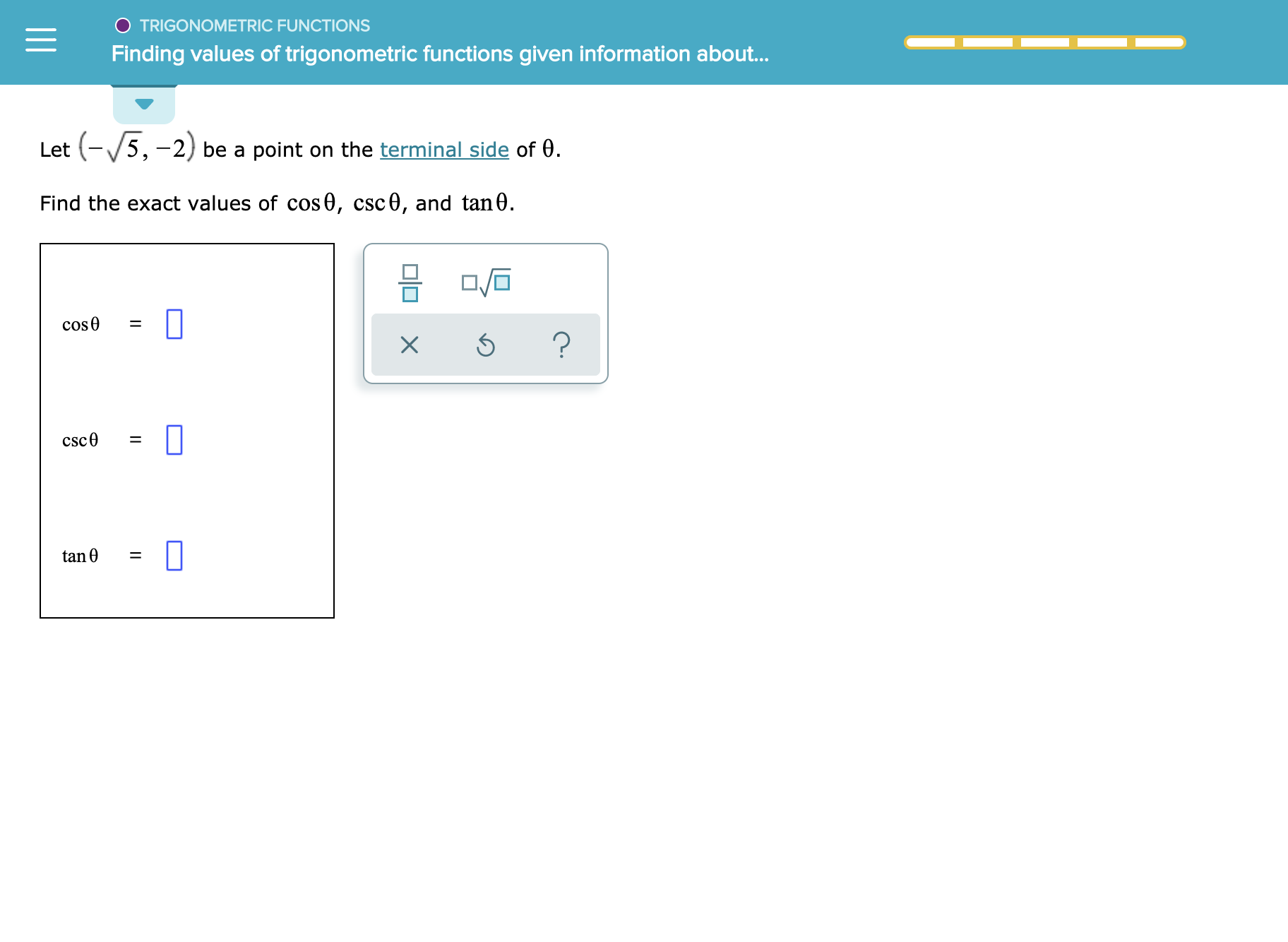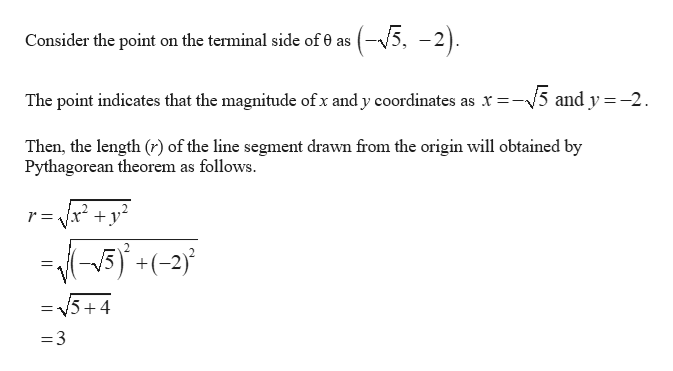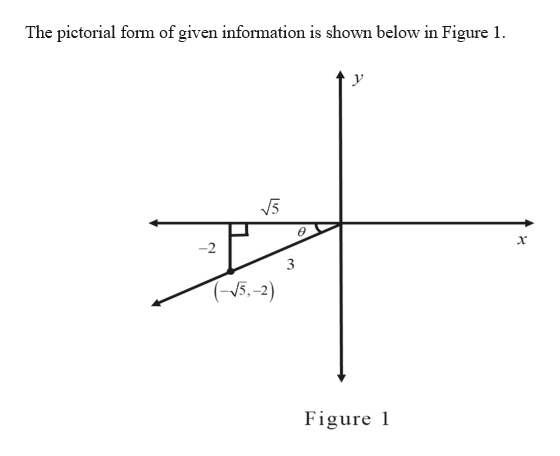# O TRIGONOMETRIC FUNCTIONSFinding values of trigonometric functions given information abou...(-5,-2) be a point on the terminal side of 0LetFind the exact values of cos0, csc0, and tan0.cose?csc0tan eX

Question
1 views

See attachmenthelp_outlineImage TranscriptioncloseO TRIGONOMETRIC FUNCTIONS Finding values of trigonometric functions given information abou... (-5,-2) be a point on the terminal side of 0 Let Find the exact values of cos0, csc0, and tan0. cose ? csc0 tan e X fullscreen
check_circle

Step 1help_outlineImage Transcriptionclose(-5, -2). Consider the point on the terminal side of 0 as The point indicates that the magnitude ofx and y coordinates as x 5 and y=-2 Then, the length () of the line segment drawn from the origin will obtained by Pythagorean theorem as follows. 5(-2 /5+ 4 =3 fullscreen
Step 2help_outlineImage TranscriptioncloseThe pictorial form of given information is shown below in Figure 1 У х -2 3 (5.-2) Figure fullscreen

### Want to see the full answer?

See Solution

#### Want to see this answer and more?

Solutions are written by subject experts who are available 24/7. Questions are typically answered within 1 hour.*

See Solution
*Response times may vary by subject and question.
Tagged in

### Other